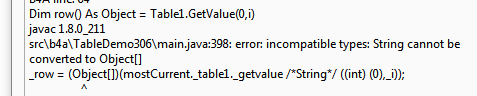Android Question[Solved]Delete table row based on value of 1st column

tcgoh

Active Member
Hi,

I have a table with empty cell in 1st column, how do i delete the row based on the 1st column,

For i = 0 To Table1.NumberOfRows -1
Dim row() As Object = Table1.GetValue(0,i)
Dim name As String = row(0)
If name = "" Then
Table1.RemoveRow(i)
Return
End If
Next

The above don't work. Any help thanks.

Computersmith64

Well-Known Member
Is the string really empty? If so you could try:
B4X:
For i = 0 To Table1.NumberOfRows -1
Dim row() As Object = Table1.GetValue(0,i)
Dim name As String = row(0)
If name.Length = 0 Then
Table1.RemoveRow(i)
Return
End If
Next

'Or if there could be spaces in it

For i = 0 To Table1.NumberOfRows -1
Dim row() As Object = Table1.GetValue(0,i)
Dim name As String = row(0)
If name.Trim.Length = 0 Then
Table1.RemoveRow(i)
Return
End If
Next

- Colin.

tcgoh

Active Member
The line
Dim row() as object = table1.getvalue(i)
Don't match as one is object vs strings ??

DonManfred

Expert
Don't match as one is object vs strings ??
do you get any error using it?

tcgoh

Active Member
yes,klaus

Expert
I would do it like this, removing all rows with an empty value in the first column:
B4X:
For i = Table1.NumberOfRows -1 To 0
Dim name As String = Table1.GetValue(0, i)
If name = "" Then
Table1.RemoveRow(i)
End If
Next
Table1.GetValue(col, row) returns a String and not an array of objects !
Table1.GetValues(row) returns an array of Strings containing the values of all columns in the given row.

tcgoh

Active Member
I would do it like this, removing all rows with an empty value in the first column:
B4X:
For i = Table1.NumberOfRows -1 To 0
Dim name As String = Table1.GetValue(0, i)
If name = "" Then
Table1.RemoveRow(i)
End If
Next
Table1.GetValue(col, row) returns a String and not an array of objects !
Table1.GetValues(row) returns an array of Strings containing the values of all columns in the given row.
Thanks Klaus,
But this code doesn't work also. No error generated and the rows are not deleted.

klaus

Expert
Sorry, it should be:

B4X:
For i = Table1.NumberOfRows - 1 To 0 Step -1
Dim name As String = Table1.GetValue(0, i)
If name = "" Then
Table1.RemoveRow(i)
End If
Next
This code works, I tested it.

Last edited:

tcgoh

Active Member
Finally get a chance to test it. It works great Thanks Klaus.

Replies
142
Views
10K
Replies
2
Views
489
Replies
1
Views
289
Replies
1
Views
204
Replies
1
Views
788